### Market Equilibrium Class 12th Introductory Microeconomics CBSE Solution

##### Question 1.Explain market equilibrium.Answer:Market equilibrium is also known as market clearing price, it refers to perfect balance in the market supply and demand, that is market equilibrium means when supply is equal to demand.When the market is at equilibrium the price of product will remain same unless some external factors change the level of supply or demand.According to market economy, there is a single price which brings demand and supply in to balance called the equilibrium price.Therefore market equilibrium is a situation in which supply of an item is exactly equal to its demand. Since there is neither surplus nor shortage in the market, the price tends to remain stable in this situation.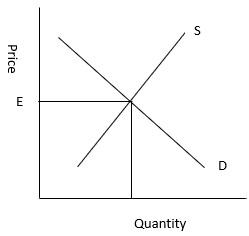Here, S is the supply curve and D is the demand curve, they intersect at price E, which is the equilibrium price.Question 2.When do we say there is excess demand for a commodity in the market?Answer:When at a given price the market demand exceeds market supply then there is excess demand for a commodity in the market.If price is below the equilibrium price then the demand will be greater than supply, due to which there will be shortage.If there is shortage, the firm will increase the prices as well as supply. With rise in price there will be movement along the demand curve and the demand will fall.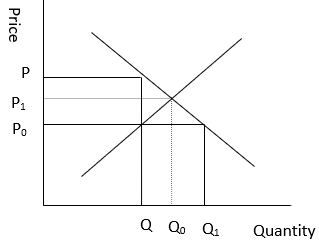In the given graph at equilibrium price P the demand was Q, now when the price falls to P0 the demand increases to Q1, which is greater than supply so there is a shortage in supply ranging from Q to Q1. To meet this demand, the price is raised from P0 to P1 due to which the demand falls from Q1 to Q0Question 3.When do we say there is excess supply for a commodity in the market?Answer:When at a given price, the supply of a commodity is greater than market demand then there is excess supply for a commodity in the market.When price will be above equilibrium price, then supply will be greater than demand it will result in surplus. To deal with the surplus, the firms will reduce price as well as supply which will increase the demand and the surplus will be eliminated.Question 4.What will happen if the price prevailing in the market is –(i) Above the equilibrium price?(ii) Below the equilibrium price?Answer:If the price prevailing in the market is above the equilibrium price –Then the demand will be less than the supply, it means a situation of excess supply will be in the marketIf the price prevailing in the market is below the equilibrium price –Then there will be more demand and less supply, it means situation of excess demand or shortage of supply.Question 5.Explain how price is determined in a perfectly competitive market with fixed number of firms.Answer:In a perfectly competitive market equilibrium price is determined by the market forces of demand and supply.Market equilibrium is determined when market demand is equal to market supply.Market demand is the total demand for a commodity by all the buyers in the market. Demand curve slopes downward due to the law of demand.Market supply is the total of supplies of a commodity by all the firms in the market, its curve slope upward due to law of supply.Equilibrium price is where the market demand curve and market supply curve intersect each other that is where market demand is equal to market supply.The schedule and graph given below will help to understand the concept -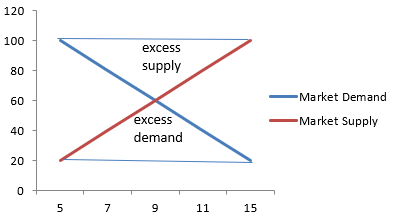The equilibrium price is Rs 9 where market demand is equal to market supplyQuestion 6.Suppose the price at which equilibrium is attained in exercise 5 is above the minimum average cost of the firms constituting the market. Now if we allow for free entry and exit of firms, how will the market price adjust to it?Answer:In the above question the equilibrium price is Rs 9, where market demand is equal to market supply at 60 units.If this equilibrium price of Rs 9 is above the minimum of average cost then it means the firm is earning supernormal profit, this situation will attract new firms in the market and with the new entrants the supply will increase.The increase in supply will result in fall in price until it becomes equal to the minimum of average cost and so the supernormal profit will be wiped out and all the firms will earn normal profit.When free entry and exit of firms is allowed equilibrium is determined by the intersection of demand curve and P is equal to minimum average cost line.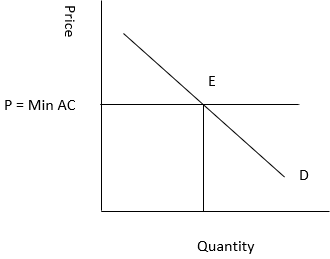Question 7.How are the equilibrium price and quantity affected when –(a) Both demand and supply curves shift in the same direction?(b) Demand and supply curves shift in opposite directions?Answer:The change in equilibrium price and quantity in following two cases are explained below –(a) Both demand and supply curves shift in the same direction?When both demand and supply curve shift in the same direction there can be six types of cases. All of them are being explained with the help of following table –(b) Demand and supply curves shift in opposite directions?When demand and supply curve shift in the opposite directions, there can be six types of cases. All of them are being explained with the help of following table –Question 8.At what level of price do the firms in a perfectly competitive market supply when free entry and exit is allowed in the market? How is equilibrium quantity determined in such a market?Answer:When free entry and exit is permitted in a perfectly competitive market the market will always supply at a price where no firm earns abnormal profit or suffers abnormal loss. Therefore the market price will be the price which is equal to the minimum average cost.The equilibrium quantity is determined at the intersection of market demand curve with price line, where price is equal to minimum average cost.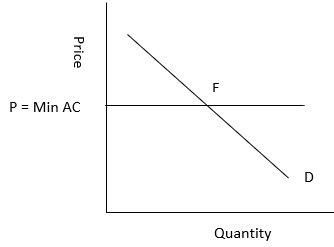Question 9.How is the equilibrium number of firms determined in a market where entry and exit is permitted?Answer:The feature of free entry and exit of firms in the market ensures that all the firms are earning normal profit, which means the market price is always equal to the minimum of LAC.No new firm will be attracted to enter the market and no existing firm will leave, if the price is equal to the minimum of LAC. Thus the number of firms is determined by the equality of price and minimum of LAC.The equilibrium number of firms in a market where free entry and exit is permitted is determined by equilibrium quantity supply per firm.Equilibrium number of firms = Equilibrium Quantity / Supply of each firmExample – Suppose the equilibrium price is P1 and equilibrium quantity is q1, Output of each firm is q1f (as all firms are identical).Equilibrium number of firms (N ) = q1/q1fQuestion 10.How are equilibrium price and quantity affected when income of the consumers(a) increase? (b) decrease?Answer:If the number of firms is fixed, when income of consumer will increase, the demand will also increase because the consumer would pay higher price but the supply will remain same, so there will be a rise in equilibrium price. As a result the demand curve will shift rightward. It will be true only in case of normal goods.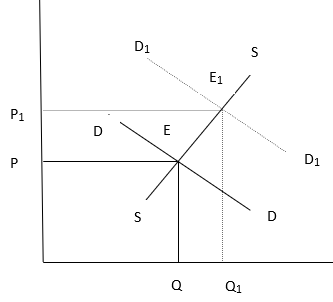Suppose, the original equilibrium price was P at point E where, supply curve SS intersected with demand curve DD. With increase in income of consumer the demand curve shifted to D1D1 and as the supply is same so the equilibrium point shifted to E1 and thus the price rises to P1If the number of firms is fixed, when there is decrease in consumer's income equilibrium price will decrease because the consumer would pay less due to less income. As a result the demand curve will shift leftward. It will be true only in case of normal goods.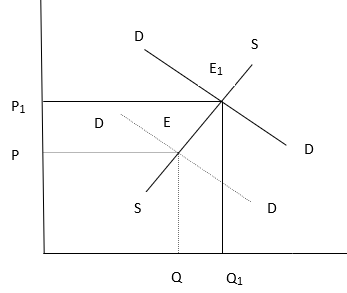Suppose, the original equilibrium price was P1 at point E1 where, supply curve SS intersected with demand curve D1D1. With decrease in income of consumer the demand curve shifted to DD and as the supply is same so the equilibrium point shifted to E and thus the price falls to P.Question 11.Using supply and demand curves, show how an increase in the price of shoes affects the price of a pair of socks and the number of pairs of socks bought and sold.Answer:Shoes and socks are complementary items, they are used together so change in price of one will affect the demand of another.The rise in price of shoes will decrease the demand of socks and vice versa.It has been further explained with help of demand schedule and graph –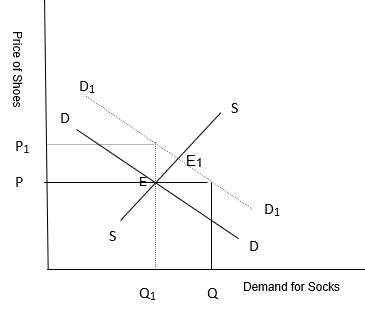When the price of socks was P the demand for socks was Q represented by demand curve DD and equilibrium point of demand and supply was E.Supply being same, when the price of shoes rises to P1 the demand falls to Q1 represented by the demand curve D1D1 and equilibrium point of demand and supply shifts to E1.Question 12.How will a change in price of coffee affect the equilibrium price of tea? Explain the effect on equilibrium quantity also through a diagram.Answer:Tea and coffee are substitute to each other so the change in price of coffee will have a direct impact on the demand for tea. Increase in price of coffee will increase the demand for tea and decrease in the price of coffee will decrease the demand of tea.Now with help of the following diagram we will explain it.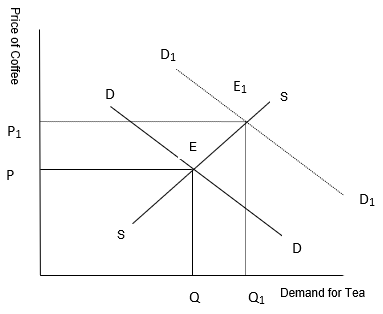Here at price P of coffee the demand for tea is Q, when the price of coffee rises to P1 the demand for tea rises to Q1 which shifts the equilibrium point from E to E1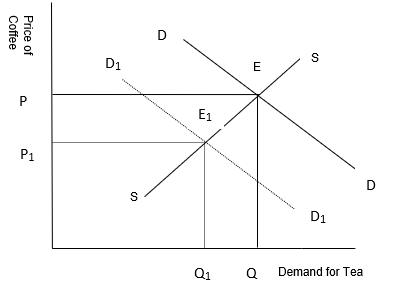Here at price P of coffee the demand for tea is Q, when the price of coffee falls to P1 the demand for tea falls to Q1 which shifts the equilibrium point from E to E1Question 13.How do the equilibrium price and quantity of a commodity change when price of input used in its production changes?Answer:a) If the price of input of a firm will increase, the cost of production will also increase, which will decrease the profit margin due to which the supply will fall. This will lead to leftward shift in the supply curve. The demand curve remaining the same, the new equilibrium will occur at E1 with higher equilibrium price P1 and lower quantity of output Q1.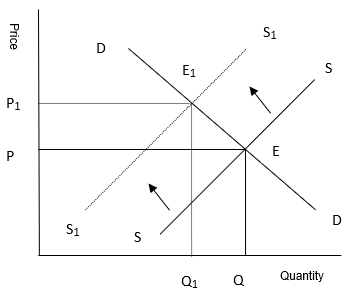b) If the price of input of a firm will decrease, the cost of production will reduce which will increase the profit margin and the firms will supply more. This will shift the supply curve rightward. Demand curve remaining the same, the new equilibrium will occur at E1 with lower equilibrium price P1 and higher quantity of output Q1.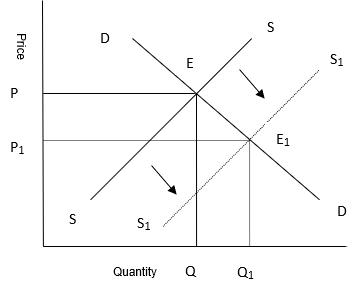Question 14.If the price of a substitute(Y) of good X increases, what impact does it have on the equilibrium price and quantity of good X?Answer:X and Y are substitute goods so the change in price of Y will have a direct impact on the demand for X. Increase in price of Y will increase the demand for X and decrease in the price of Y will decrease the demand of X.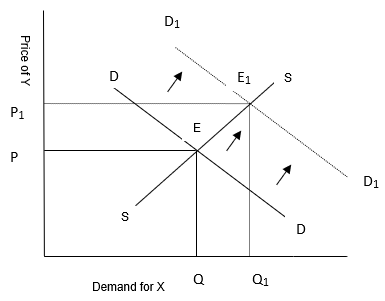Question 15.Compare the effect of shift in demand curve on the equilibrium when the number of firms in the market is fixed with the situation when entry-exit is permitted.Answer:Tea and coffee are substitute to each other so the change in price of coffee will have a direct impact on the demand for tea. Increase in price of coffee will increase the demand for tea and decrease in the price of coffee will decrease the demand of tea.Question 16.Explain through a diagram the effect of a rightward shift of both the demand and supply curves on equilibrium price and quantity.Answer:The effect of rightward shift of both demand and supply curve on equilibrium price and quantity can be explained in following three manners –1. Where demand and supply increase in same proportion - In this case the equilibrium price will remain unchanged.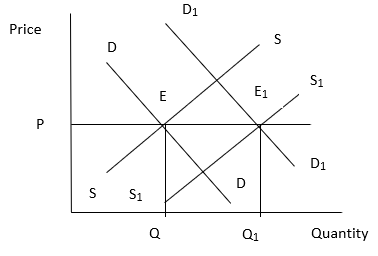In the given graph the original demand curve shifts from DD to D1D1 and supply curve from SS to S1 S1 due to which the equilibrium point shifts from E to E1 but the equilibrium price is same i.e. P.2. Where demand increases more than the increase in supply - In this case the equilibrium price will rise.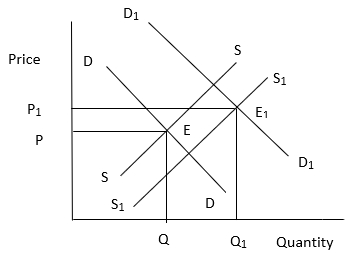In the given graph the original demand curve shifts from DD to D1D1 and supply curve from SS to S1 S1. The increase in demand curve is more than that in the supply curve due to which the equilibrium point shifts from E to E1 which increases the equilibrium price to P1.3. Where demand increases less than the increase in supply - In this case the equilibrium price will fall.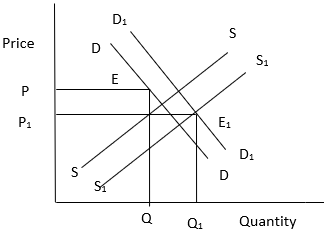In the given graph the original demand curve shifts from DD to D1D1 and supply curve from SS to S1 S1. The increase in supply curve is more than that in the demand curve due to which the equilibrium point shifts from E to E1 which decreases the equilibrium price to P1.Question 17.In what respect do the supply and demand curves in the labour market differ from those in the goods market?Answer:The difference between supply and demand curves in labour market than that in the goods market are stated belowa) In goods market the demand is made by consumers or household while in labour market demand is made by firms.b) In goods market the supply of goods is made by firms where as in labour market the supply of labour is made by households.c) In goods market firms act as suppliers where is in labour market household acts as suppliers.Question 18.How is the optimal amount of labour determined in a perfectly competitive market?Answer:A profit maximising firm will employ labour up to the point where extra wages paid to the last unit of labour is equal to the additional benefit earned by employing that unit of labour.That is when marginal cost of labour is equal to marginal benefit by labour or wage rate is equal to marginal revenue product.The optimal amount of labour is determined in a perfectly competitive market asVMPL = WWhere,VMPL = Value of Marginal Product of LabourW = Wage rate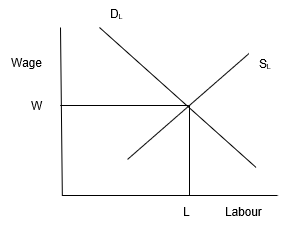Question 19.How is the wage rate determined in a perfectly competitive labour market?Answer:The wage rate in labour market is determined by the intersection of demand for and supply of labour just like in goods market. The rate at which the demand for labour and supply of labour will be at equilibrium will be the wage rate.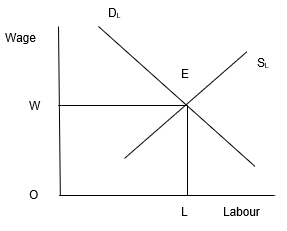Here, OW will be the wage rate in a perfectly competitive market, where supply curve of labour intersects the demand curve of labour.Question 20.Can you think of any commodity on which price ceiling is imposed in India? What may be the consequence of price-ceiling?Answer:Price ceiling means deciding the lower prices as compared to the market price of goods. In India there are several goods on which government has imposed price ceiling so as to keep them available within the reach of the people below poverty line such as kerosene oil, sugar, wheat, rice, etc.The consequences of price ceiling as stated below –a) Excess demand - Due to artificially imposed prices, which is lower than the equilibrium price will lead to excess demandb) Fixed quota - The consumer gets a fixed quantity of goods which is often less than their individual requirement due to which the consumer remains unsatisfied.c) Inferior goods - Often it has been found that the goods that are rationed are inferior in quality and adulterated.d) Black marketing - The needs of a consumer remains unfulfilled due to quota fixed by government and such consumers are ready to pay higher prices for additional quantity which leads to black marketing and artificial shortage in market.Question 21.A shift in demand curve has a larger effect on price and smaller effect on quantity when the number of firms is fixed compared to the situation when free entry and exit is permitted. Explain.Answer:In short run the number of firms is fixed where as in long run the number of firms is not fixed.Now with help of following two graphs we will explain the effect in short run market –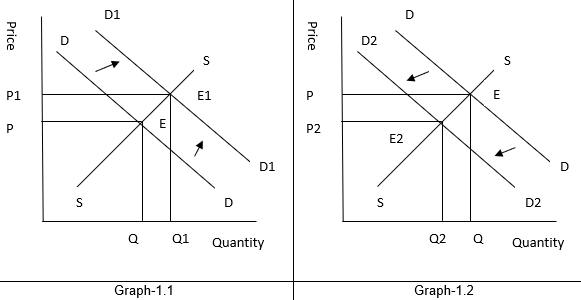In short run, when number of firms is fixed –Graph 1.1 - DD represents the demand curve and E is the initial equilibrium, where demand and supply intersect each other.Now when the demand increases the demand curve shifts to D1D1, new equilibrium E1 arises where supply curve and demand curve intersect each other at price P1 and equilibrium quantity Q1. This means both equilibrium price and quantity rises.Graph 1.2 - DD represents the demand curve and E is the initial equilibrium, where demand and supply intersect each other.Now when the demand decreases the demand curve shifts to D2D2, new equilibrium E2 arises where supply curve and demand curve intersect each other at price P2 and equilibrium quantity Q2. This means both equilibrium price and quantity falls.Now with help of following two graphs we will explain the effect in long run market –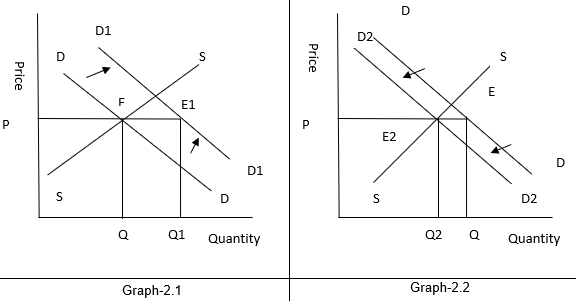In long run, when number of firms is not fixed, but free entry and exit is allowed –Graph 2.1 - DD represents the demand curve and E is the initial equilibrium, where P = Min ACNow when the demand increases the demand curve shifts to D1D1, new equilibrium E1 arises where price is same and equilibrium quantity is Q1. This means equilibrium price remains same but equilibrium quantity rises.Graph 2.2 - DD represents the demand curve and E is the initial equilibrium, where P = Min AC.Now when the demand decreases the demand curve shifts to D2D2, new equilibrium E2 arises where price is same and equilibrium quantity is Q2. This means equilibrium price remains same but equilibrium quantity falls.Question 22.Suppose the demand and supply curve of commodity X in a perfectly competitive market are given by:qD = 700 – pqS = 500 + 3p for p ≥ 15= 0 for 0 ≤ p < 15Assume that the market consists of identical firms. Identify the reason behind the market supply of commodity X being zero at any price less than Rs 15. What will be the equilibrium price for this commodity? At equilibrium, what quantity of X will be produced?Answer:Given –qD = 700 – pqS = 500 + 3p for p ≥ 15= 0 for 0 ≤ p < 15From the given data we can see that the market supply is zero for the price range Rupees 0 to Rupees 15 because it is less than the minimum of average variable cost, so no firm will produce any positive level of output and the market supply curve will be zero.At equilibrium, qD = qS700 – p = 500 + 3p200 = 4pP = 50Therefore, the equilibrium price is Rs 50.At equilibrium price, the equilibrium quantity will beqS = 500 + 3p= 500 + 3 (50)= 650 units.Therefore, the equilibrium quantity is 650 units.Question 23.Considering the same demand curve as in exercise 22, now let us allow for free entry and exit of the firms producing commodity X. Also assume the market consists of identical firms producing commodity X. Let the supply curve of a single firm be explained as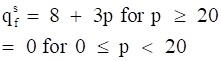(a) What is the significance of p = 20?(b) At what price will the market for X be in equilibrium? State the reason for your answer.(c) Calculate the equilibrium quantity and number of firms.Answer:(a) What is the significance of p = 20?For the price between zero to 20 no form is going to produce anything because the price is below minimum of LAC, so at the price of rupees 20 the price line is equal to minimum of LAC.(b) At what price will the market for X be in equilibrium? State the reason for your answer.As free entry and exit of firms is allowed the minimum of AVC is at Rs 20 which is also the equilibrium price because in long run all firms earn zero economic profit. So at any price lower than Rs 20 the firm will move out of the market.(c) Calculate the equilibrium quantity and number of firms.qs = 8 + 3p= 8 + 3 (20)= 68 unitsqD = 700 – p= 700 – 20= 680 unitsNo. Of Firms = qD / qs = 10Question 24.Suppose the demand and supply curves of salt are given by:qD = 1,000 – p qS = 700 + 2p(a) Find the equilibrium price and quantity.(b) Now suppose that the price of an input used to produce salt has increased so that the new supply curve isqS = 400 + 2pHow does the equilibrium price and quantity change? Does the change conform to your expectation?(c) Suppose the government has imposed a tax of Rs 3 per unit of sale of salt. How does it affect the equilibrium price and quantity?Answer:(a) Find the equilibrium price and quantity.At equilibrium point qD = qS1000 – p = 700 + 2p300 = 3pp = 100Therefore, equilibrium price is Rs 100qD = 1,000 – p= 1000 – 100= 900 unitsTherefore, equilibrium quantity is 900 units(b) Now suppose that the price of an input used to produce salt has increased so that the new supply curve isqS = 400 + 2pHow does the equilibrium price and quantity change? Does the change conform to your expectation?Now for equilibrium price1000 – p = 400 + 2p600 = 3pp = 200Therefore, equilibrium price is Rs 100qs = 400 + 2p= 800Therefore, equilibrium quantity is 800 unitsThe equilibrium quantity has decreased because the cost of production has increased which will shift the marginal cost curve leftward and move the supply curve towards the left, the leftward shift in the supply curve will result in increase in equilibrium price and fall in equilibrium quantity.(c) Suppose the government has imposed a tax of Rs 3 per unit of sale of salt. How does it affect the equilibrium price and quantity?The imposition of tax of Rs 3 per unit of salt sold will raise the cost of producing salt. Thiswill shift the supply curve leftwards and the quantity supplied equation will becomeqs= 700 + 2 (p - 3)At equilibrium point qD = qS1000 – p = 700 + 2 (p – 3)1000 – p = 700 + 2p - 61000 – 694 = 3pp = 102Therefore, equilibrium price is Rs 102qd = 1000 – p= 1000 – 102= 898Therefore, equilibrium quantity is 898 unitsQuestion 25.Suppose the market determined rent for apartments is too high for common people to afford. If the government comes forward to help those seeking apartments on rent by imposing control on rent, what impact will it have on the market for apartments?Answer:If the government imposes price ceiling by controlling the rent that is fixing the maximum price that can be charged as the rent of apartment it will decline the equilibrium price due to• Excess demand of apartment and• Black marketing by Builders

PDF FILE TO YOUR EMAIL IMMEDIATELY PURCHASE NOTES & PAPER SOLUTION. @ Rs. 50/- each (GST extra)

HINDI ENTIRE PAPER SOLUTION

MARATHI PAPER SOLUTION

SSC MATHS I PAPER SOLUTION

SSC MATHS II PAPER SOLUTION

SSC SCIENCE I PAPER SOLUTION

SSC SCIENCE II PAPER SOLUTION

SSC ENGLISH PAPER SOLUTION

SSC & HSC ENGLISH WRITING SKILL

HSC ACCOUNTS NOTES

HSC OCM NOTES

HSC ECONOMICS NOTES

HSC SECRETARIAL PRACTICE NOTES

# 2019 Board Paper Solution

HSC ENGLISH SET A 2019 21st February, 2019

HSC ENGLISH SET B 2019 21st February, 2019

HSC ENGLISH SET C 2019 21st February, 2019

HSC ENGLISH SET D 2019 21st February, 2019

SECRETARIAL PRACTICE (S.P) 2019 25th February, 2019

HSC XII PHYSICS 2019 25th February, 2019

CHEMISTRY XII HSC SOLUTION 27th, February, 2019

OCM PAPER SOLUTION 2019 27th, February, 2019

HSC MATHS PAPER SOLUTION COMMERCE, 2nd March, 2019

HSC MATHS PAPER SOLUTION SCIENCE 2nd, March, 2019

SSC ENGLISH STD 10 5TH MARCH, 2019.

HSC XII ACCOUNTS 2019 6th March, 2019

HSC XII BIOLOGY 2019 6TH March, 2019

HSC XII ECONOMICS 9Th March 2019

SSC Maths I March 2019 Solution 10th Standard11th, March, 2019

SSC MATHS II MARCH 2019 SOLUTION 10TH STD.13th March, 2019

SSC SCIENCE I MARCH 2019 SOLUTION 10TH STD. 15th March, 2019.

SSC SCIENCE II MARCH 2019 SOLUTION 10TH STD. 18th March, 2019.

SSC SOCIAL SCIENCE I MARCH 2019 SOLUTION20th March, 2019

SSC SOCIAL SCIENCE II MARCH 2019 SOLUTION, 22nd March, 2019

XII CBSE - BOARD - MARCH - 2019 ENGLISH - QP + SOLUTIONS, 2nd March, 2019

# HSCMaharashtraBoardPapers2020

(Std 12th English Medium)

HSC ECONOMICS MARCH 2020

HSC OCM MARCH 2020

HSC ACCOUNTS MARCH 2020

HSC S.P. MARCH 2020

HSC ENGLISH MARCH 2020

HSC HINDI MARCH 2020

HSC MARATHI MARCH 2020

HSC MATHS MARCH 2020

# SSCMaharashtraBoardPapers2020

(Std 10th English Medium)

English MARCH 2020

HindI MARCH 2020

Hindi (Composite) MARCH 2020

Marathi MARCH 2020

Mathematics (Paper 1) MARCH 2020

Mathematics (Paper 2) MARCH 2020

Sanskrit MARCH 2020

Sanskrit (Composite) MARCH 2020

Science (Paper 1) MARCH 2020

Science (Paper 2)

Geography Model Set 1 2020-2021

MUST REMEMBER THINGS on the day of Exam

Are you prepared? for English Grammar in Board Exam.

Paper Presentation In Board Exam

How to Score Good Marks in SSC Board Exams

Tips To Score More Than 90% Marks In 12th Board Exam

How to write English exams?

How to prepare for board exam when less time is left

How to memorise what you learn for board exam

No. 1 Simple Hack, you can try out, in preparing for Board Exam

How to Study for CBSE Class 10 Board Exams Subject Wise Tips?

JEE Main 2020 Registration Process – Exam Pattern & Important Dates

NEET UG 2020 Registration Process Exam Pattern & Important Dates

How can One Prepare for two Competitive Exams at the same time?

8 Proven Tips to Handle Anxiety before Exams!